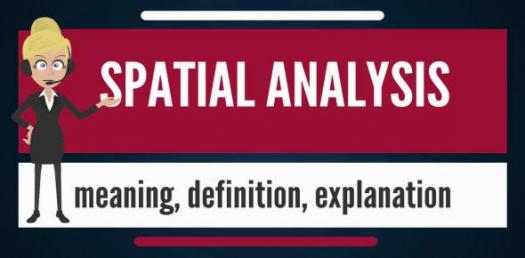# Spatial Analysis Trivia Quiz

10 Questions | Total Attempts: 160SettingsThe Spatial analysis includes different techniques used to study entities using different useful properties, either topological or geometrical or the geographic property of the entity. What do you know about Spatial analysis? Try this quiz.

• 1.
Which of these is Spatial analysis called?
• A.

Spatial clarification

• B.

Spatial differentiation

• C.

Spatial statistics

• D.

Spatial algorithm

• 2.
What is the full meaning of MPS?
• A.

Migration and population statistics

• B.

Multiple-point Geostatistics

• C.

Multiple Statistics

• D.

Multiple Partial Statistics

• 3.
Which of these is not an advanced operation of Spatial analysis?
• A.

Geovisualisation

• B.

Surface analysis

• C.

Power analysis

• D.

Network analysis

• 4.
Which of these have not contributed to the rise of Spatial analysis in mordern times?
• A.

Language development

• B.

Computer

• C.

Economics

• D.

Biology

• 5.
When can we say a mathematical space exist?
• A.

When there is an observation and quantitative measure of the attributes

• B.

When there is an observation and no quantitative measure of the attributes

• C.

When an observation is not clearly stated

• D.

When the attributes of the observation is unbalanced

• 6.
Which type of mathematical space is Spatial analysis interested in?
• A.

Formular distinction

• B.

Horizon mapping

• C.

Computer space

• D.

Geographical space

• 7.
Which of these is not a type of Spatial analysis?
• A.

Spatial interpolation

• B.

Spatial acceleration

• C.

Spatial regression

• D.

Spatial autocorrelation

• 8.
Which of these is a Spatial simulation method?
• A.

Character animation

• B.

Football agency

• C.

Agent based modeling

• D.

Studio management

• 9.
What other term is given to Spatial interaction?
• A.

Differential arrangements

• B.

Gravity models

• C.

Symbiosis

• D.

Gravity drops

• 10.
What does factor analysis depend on?
• A.

Correspondence Analysis

• B.

Distance between observation

• C.

Principal Component Analysis

• D.

Discriminant Analysis

Related TopicsBack to top